Next: Preservation of the RPA Up: Theoretical WT predictions Previous: Theoretical WT predictions

## Evolution equations for the PDF's, moments and spectrum.

In  the following equation for for the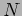-mode PDF was obtained for the four-wave systems,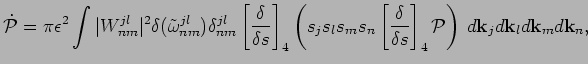(16)

where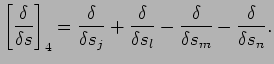(17)

Here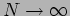limit has already been taken and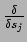means the variational derivative. Using this equation, one can prove that RPA property holds over the nonlinear time, i.e. the-mode PDF remains of the product factorized form with accuracy sufficient for the WT closures to work . Using RPA, we get for the one-point marginals ,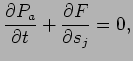(18)

with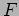is a probability flux in the s-space,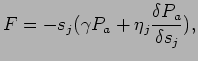(19)

where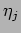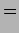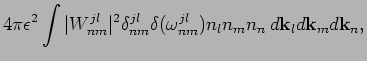(20)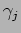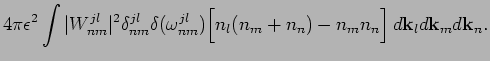(21)

Here we introduced the wave-action spectrum,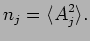(22)

From (14) we get the following equation for the moments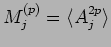: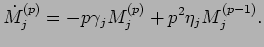(23)

which, for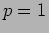gives the standard wave kinetic equation (WKE),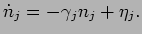(24)Next: Preservation of the RPA Up: Theoretical WT predictions Previous: Theoretical WT predictions
Dr Yuri V Lvov 2007-01-16